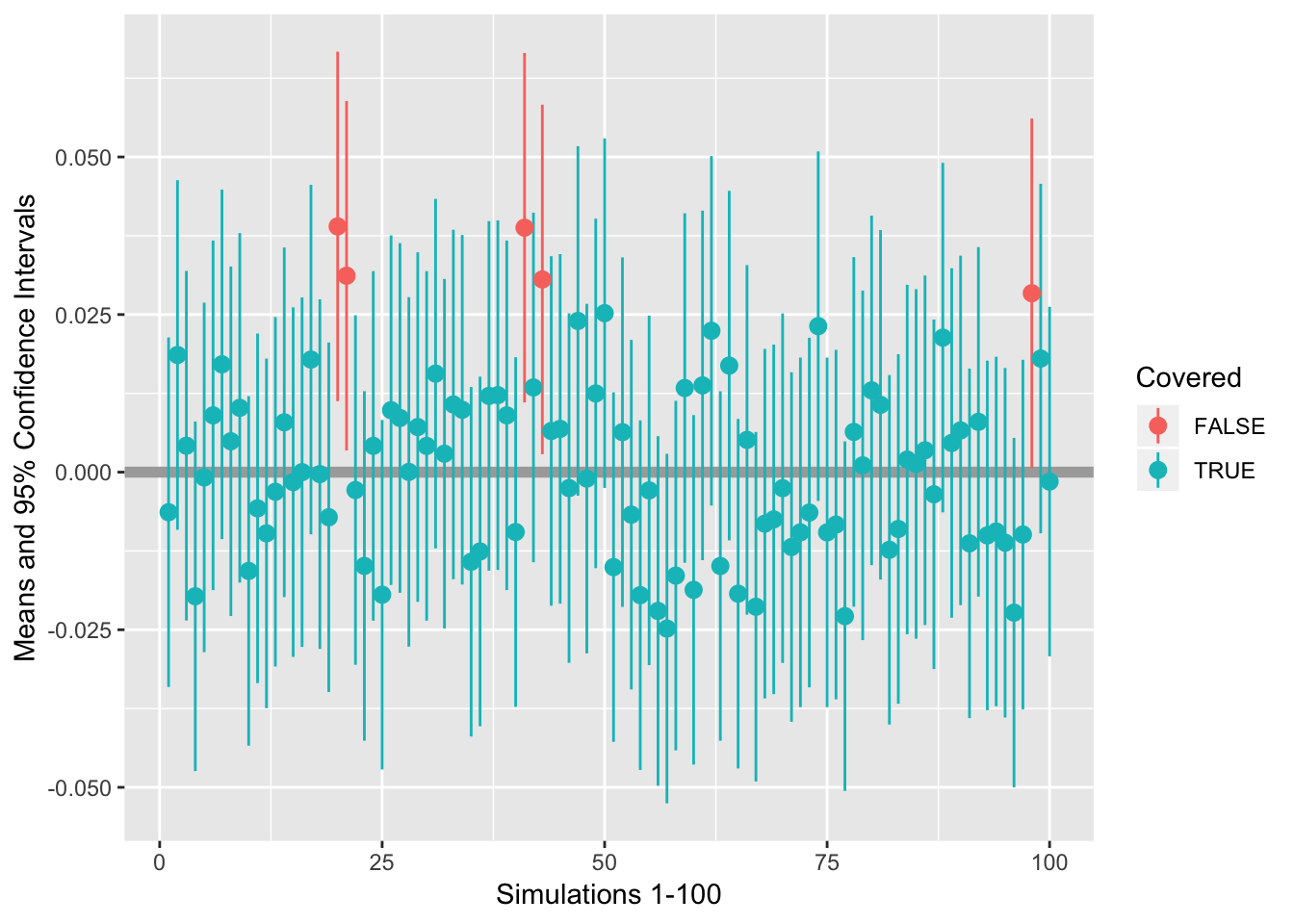An Exercise in using rmarkdown to adopt a reproducible workflow

We start by fixing the random seed. Otherwise this whole exercise is redundant.

set.seed(1234)

Then, we draw 100 samples from a standard normal distribution, i.e. a distribution with $$\mu=0$$ and $$\sigma^2=1$$, such that for any drawn sample $$X$$ we could write $$X \sim \mathcal{N}(0, 1)$$. No specification about the size of the samples is explicitly requested, so for computational reasons a mere 5000 cases would suffice to obtain a detailed approximation.

library(plyr)
samples <- rlply(100, rnorm(5000, mean = 0, sd = 1))

We use the plyr::rlply() function to draw the 100 samples and return the resulting output as a list. Further, we extract the following sources of information for each sample:

• the sample mean $$\bar{x}$$.
• the absolute bias: i.e. $$|\bar{x} - \mu|$$, which would be $$\bar{x}$$ in this case.
• the standard error of the mean: $$SE = \sigma/\sqrt{n}$$, where $$\sigma$$ is the known population standard deviation and $$n$$ is the number of observations in the sample.
• the upper- and lower bounds of the 95% confidence interval about the sample mean:
info <- function(x){
M <- mean(x)
DF <- length(x) - 1
SE <- 1 / sqrt(length(x))
INT <- qt(.975, DF) * SE
return(c(M, M - 0, SE, M - INT, M + INT))
}
format <- c("Mean" = 0, "Bias" = 0, "Std.Err" = 0, "Lower" = 0, "Upper" = 0)

We can then proceed by creating a piped process. The following code is written with the pipe functionality from package magrittr - FYI: dplyr adopted magrittr, so dplyr would also work here.

require("magrittr")
results <- samples %>%
vapply(., info, format) %>%
t()

Because object samples is a list, we can execute funtion vapply() to obtain a numerical object with the results of function info(). vapply() allows you to return output to a pre-defined format. Function $$t()$$ is here used to obtain the transpose of vapply()’s return - the resulting object has all information in the columns.

To create an indicator for the inclusion of the population value $$\mu=0$$ in the confidence interval, we can add the following coverage column cov to the data:

results <- results %>%
as.data.frame() %>%
mutate(Covered = Lower < 0 & 0 < Upper)

Converting the numerical object to an object of class data.frame allows for a more convenient calling of elements. Now we can simply take the column means over dataframe results to obtain the average of the estimates returned by info().

colMeans(results)
##         Mean         Bias      Std.Err        Lower        Upper
##  0.001341244  0.001341244  0.014142136 -0.026383545  0.029066033
##      Covered
##  0.950000000

We can see that 95 out of the 100 samples cover the population value.

To identify the samples for which the population value is not covered, we can use column cov as it is already a logical evaluation.

table <- results[!resultsCovered, ] To present this info as a table, package kableExtra is a wonderful extension to use with both rmarkdown and $$\LaTeX$$. require(knitr) require(kableExtra) kable(table) Mean Bias Std.Err Lower Upper Covered 20 0.0389823 0.0389823 0.0141421 0.0112575 0.0667071 FALSE 21 0.0311591 0.0311591 0.0141421 0.0034343 0.0588839 FALSE 41 0.0387800 0.0387800 0.0141421 0.0110552 0.0665048 FALSE 43 0.0305673 0.0305673 0.0141421 0.0028425 0.0582921 FALSE 98 0.0283847 0.0283847 0.0141421 0.0006599 0.0561094 FALSE kable(table, "html") %>% kable_styling(bootstrap_options = c("striped", "hover", "condensed"), full_width = F, position = "float_right") Mean Bias Std.Err Lower Upper Covered 20 0.0389823 0.0389823 0.0141421 0.0112575 0.0667071 FALSE 21 0.0311591 0.0311591 0.0141421 0.0034343 0.0588839 FALSE 41 0.0387800 0.0387800 0.0141421 0.0110552 0.0665048 FALSE 43 0.0305673 0.0305673 0.0141421 0.0028425 0.0582921 FALSE 98 0.0283847 0.0283847 0.0141421 0.0006599 0.0561094 FALSE For an even more flexible presentation of tabulated results, the graphical parameters for kable() can be changed. For example, the following code renders a table that does not span the width of the page, is in a right-aligned floating container and has some visually pleasing aesthetics, like striping, hovering (mouse pointer) and is somewhat condensed. However, you need to pay attention to the final result. Justified floats have a tendency to mess up the ‘natural’ flow of the text, unless the body of text is sufficiently large. For example, this whole paragraph serves that purpose: to create a suffiently large body of text. To create a graph that would serve the purpose of the exercise, one could think about the following graph: require(ggplot2) limits <- aes(ymax = resultsUpper, ymin = results\$Lower)
ggplot(results, aes(y=Mean, x=1:100, colour = Covered)) +
geom_hline(aes(yintercept = 0), color = "dark grey", size = 2) +
geom_pointrange(limits) +
xlab("Simulations 1-100") +
ylab("Means and 95% Confidence Intervals")End of exercise 5